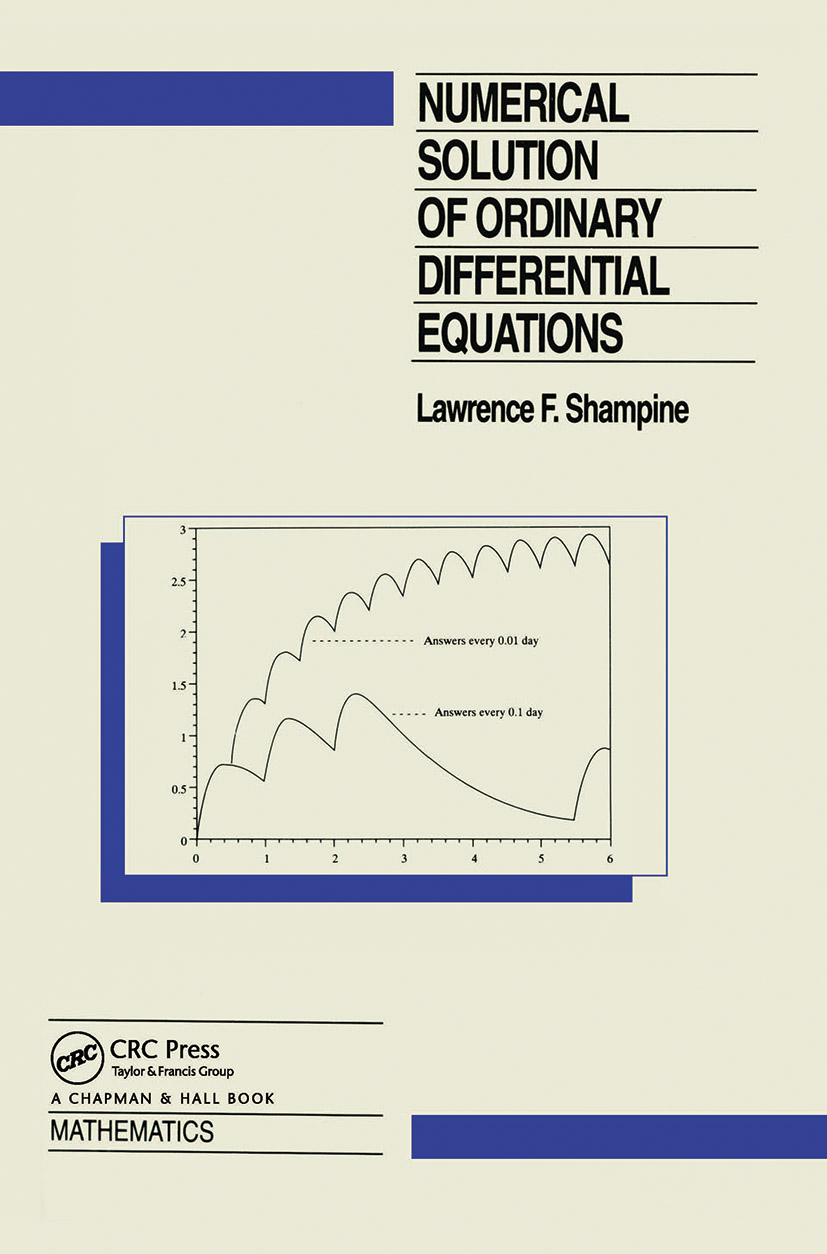# Numerical Solution of Ordinary Differential Equations

## 1st Edition

Chapman and Hall/CRC

632 pages

Hardback: 9780412051517
pub: 1994-03-01
\$195.00
x
eBook (VitalSource) : 9780203745328
pub: 2018-10-24
from \$28.98

FREE Standard Shipping!

### Description

This book is an introduction to the numerical solution of the initial value problem for a system of ordinary differential equations (ODEs). It describes how typical problems can be formulated in a way that permits their solution with standard codes.

The mathematical problem: discrete variable methods

The computational problem: basic methods

Convergence and stability: stability for large step sizes

Error estimation and control: stiff problems

Problems

References

Appendix

Some mathematical tools

Index